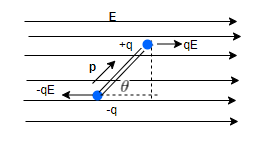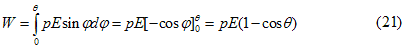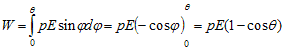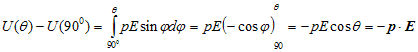# Potential Energy of a electric dipole

## Work done in rotating an electric dipole in an electric field• Consider a dipole placed in a uniform electric field and it is in equilibrium position. If we rotate this dipole from its equllibrium position , work has to be done.
• Suppose electric dipole of moment p is rotated in uniform electric field E through an angle θ from its equilibrium position. Due to this rotation couple acting on dipole changes.
• If at any instant dipole makes an angle φ with uniform electric field then torque acting on dipole is
Γ=pEsinφ                                                    (19)
again work done in rotating this dipole through an infitesimaly small angle dφ is
dW=torque x angular displacement
=pEsinφdφ
• Total work done in rotating the dipole through an angle θfrom its equilibrium position isThis is the required formula for work done in rotating an electric dipole placed in uniform electric field through an angle θ from its equilibrium position.

## Potential energy of dipole placed in uniform electric field

• Again consider equation 20 which gives the work done in rotating electric dipole through an infinetesimly small angle dφ is
dW=pEsinφdφ
which is equal to the change in potential energy of the system
dW=dU=pEsinφdφ                                                    (22)
• If angle dφ is changed from 900 to θ then in potential energy would be• We have choosen the value of φgoing from π/2 to θ because at π/2 we can take potential energy to be zero (axis of dipole is perpandicular to the field). Thus U(900)=0 and above equation becomesQuestion 1
An electric diple of length 2 cm is placed with its axis making an angle of 60° to a uniform electric field $10^5$ N/C. If its experience a torque of $8 \sqrt 3$ Nm, Find the following
(a) Magnitude of the charge on the dipole
(b) Potential energy of the dipole
Solution
(a)Let q be the charge,then
$p=q \times .02=.02 q$
Now torque is given by
$\tau = pE sin \theta$
$8 \sqrt 3=.02 q \times 10^5 \frac {\sqrt 3}{2}$
or $q=8 \times 10^{-3}$ C
(b) Now potential energy is defined as
$U=-pE cos \theta = - 8 \times 10^{-3} \times .02 \times 10^5 \times \frac {1}{2} = -8 \ J$

Question 2
A diple is placed in a uniform Electric Field E ,its potential energy will be minimum when the angle between its axis and field is
(a) $\pi$
(b) $\frac {\pi }{2}$
(c) $2 \pi$
(d) zero
Solution
$U=-pE cos \theta$
At $\theta =0$
$U= -pE$
So potential energy is minimum when axis is parallel to the Electric field
Question 3
An electric dipole of moment p is placed normal to the lines of force of electric intensity E, then the work done in deflecting it through an angle of 180° is
(a) pE
(b)2pE
(c)-2pE
(d)Zero
Solution
(d)